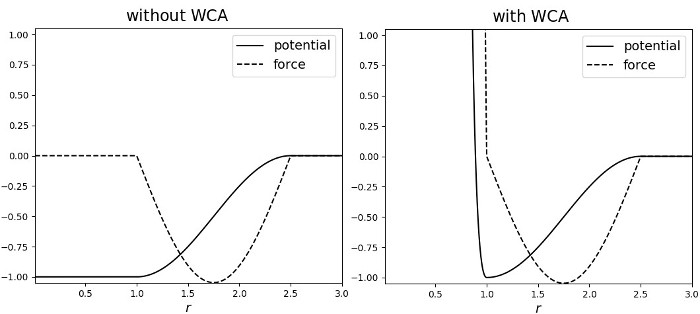$$\renewcommand{\AA}{\text{Å}}$$

# pair_style cosine/squared command

## Syntax

pair_style cosine/squared cutoff

• cutoff = global cutoff for cosine-squared interactions (distance units)

pair_coeff i j eps sigma
pair_coeff i j eps sigma cutoff
pair_coeff i j eps sigma wca
pair_coeff i j eps sigma cutoff wca

• i,j = a particle type

• eps = interaction strength, i.e. the depth of the potential minimum (energy units)

• sigma = distance of the potential minimum from 0

• cutoff = the cutoff distance for this pair type, if different from global (distance units)

• wca = if specified a Weeks-Chandler-Andersen potential (with eps strength and minimum at sigma) is added, otherwise not

## Examples

pair_style cosine/squared 3.0
pair_coeff * * 1.0 1.3
pair_coeff 1 3 1.0 1.3 2.0
pair_coeff 1 3 1.0 1.3 wca
pair_coeff 1 3 1.0 1.3 2.0 wca


## Description

Style cosine/squared computes a potential of the form

$\begin{split}E = \begin{cases} -\epsilon& \quad r < \sigma \\ -\epsilon\cos\left(\frac{\pi\left(r - \sigma\right)}{2\left(r_c - \sigma\right)}\right)^2&\quad \sigma \leq r < r_c \\ 0& \quad r \geq r_c \end{cases}\end{split}$

between two point particles, where ($$\sigma, -\epsilon$$) is the location of the (rightmost) minimum of the potential, as explained in the syntax section above.

This potential was first used in (Cooke) for a coarse-grained lipid membrane model. It is generally very useful as a non-specific interaction potential because it is fully adjustable in depth and width while joining the minimum at (sigma, -epsilon) and zero at (cutoff, 0) smoothly, requiring no shifting and causing no related artifacts, tail energy calculations etc. This evidently requires cutoff to be larger than sigma.

If the wca option is used then a Weeks-Chandler-Andersen potential (Weeks) is added to the above specified cosine-squared potential, specifically the following:

$E = \epsilon \left[ \left(\frac{\sigma}{r}\right)^{12} - 2\left(\frac{\sigma}{r}\right)^6 + 1\right] , \quad r < \sigma$

In this case, and this case only, the $$\sigma$$ parameter can be equal to cutoff ($$\sigma =$$ cutoff) which will result in ONLY the WCA potential being used (and print a warning), so the minimum will be attained at (sigma, 0). This is a convenience feature that enables a purely repulsive potential to be used without a need to define an additional pair style and use the hybrid styles.

The energy and force of this pair style for parameters epsilon = 1.0, sigma = 1.0, cutoff = 2.5, with and without the WCA potential, are shown in the graphs below:## Mixing, shift, table, tail correction, restart, rRESPA info

Mixing is not supported for this style.

The shift, table and tail options are not relevant for this style.

This pair style writes its information to binary restart files, so pair_style and pair_coeff commands do not need to be specified in an input script that reads a restart file.

These pair styles can only be used via the pair keyword of the run_style respa command. They do not support the inner, middle, outer keywords.

## Restrictions

The cosine/squared style is part of the EXTRA-PAIR package. It is only enabled if LAMMPS is build with that package. See the Build package page for more info.

## Default

none

(Cooke) “Cooke, Kremer and Deserno, Phys. Rev. E, 72, 011506 (2005)”

(Weeks) “Weeks, Chandler and Andersen, J. Chem. Phys., 54, 5237 (1971)”